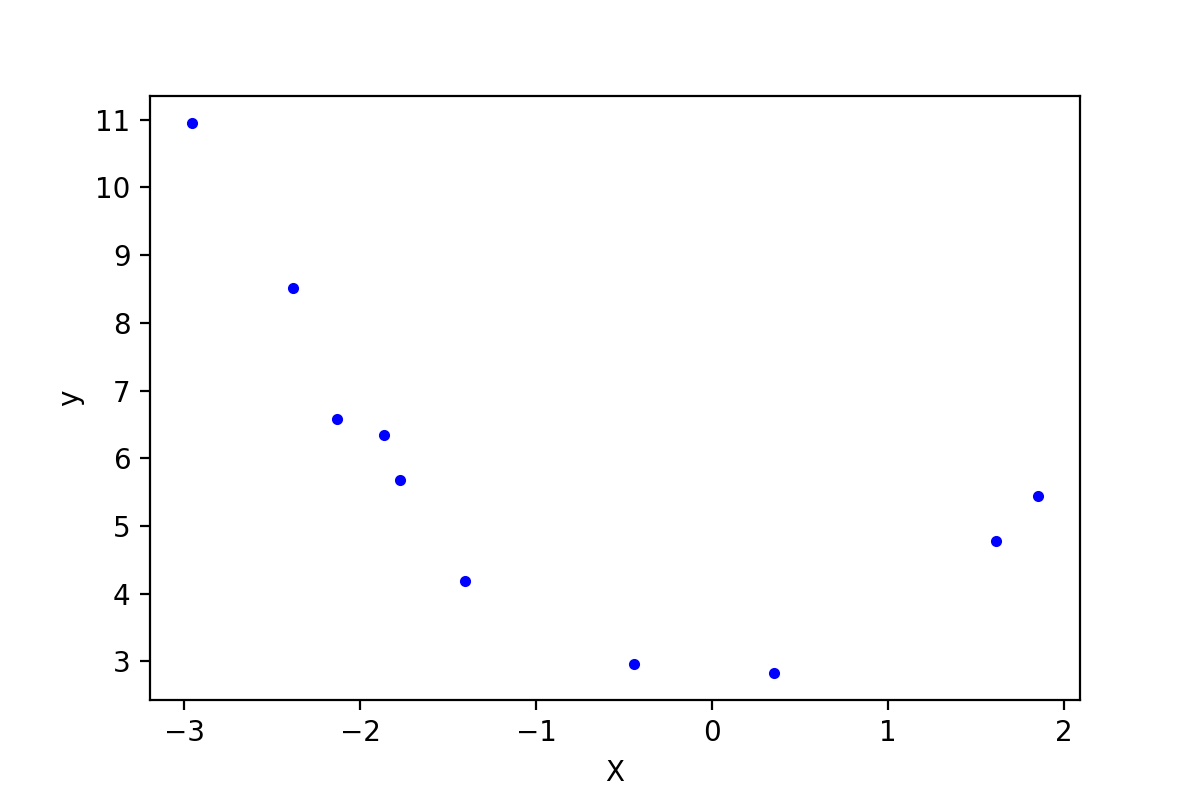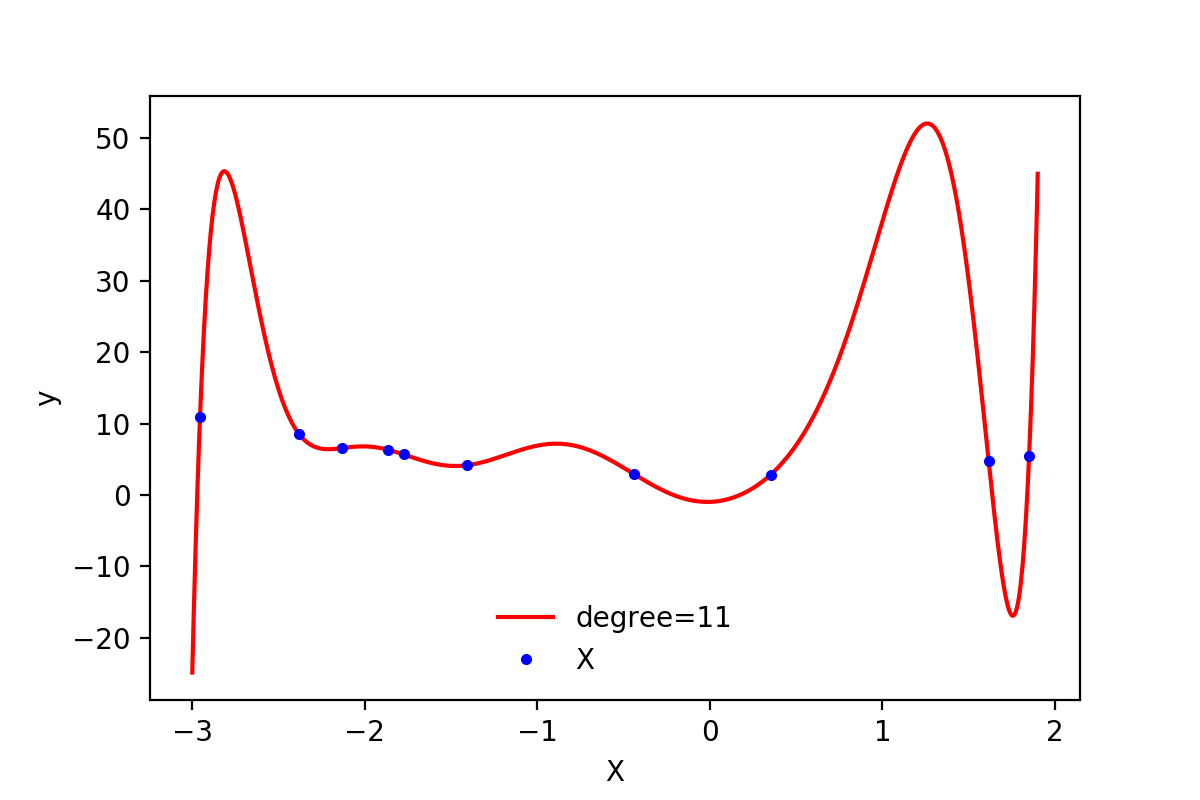# 【机器学习】多项式回归

：在上一篇的一般线性回归中，使用的假设函数是一元一次方程，也就是二维平面上的一条直线。但是很多时候可能会遇到直线方程无法很好的拟合数据的情况，这个时候可以尝试使用多项式回归。多项式回归中，加入了特征的更高次方（例如平方项或立方项），也相当于增加了模型的自由度，用来捕获数据中非线性的变化。添加高阶项的时候，也增加了模型的复杂度。随着模型复杂度的升高，模型的容量以及拟合数据的能力增加，可以进一步降低训练误差，但导致过拟合的风险也随之增加。### 0. 多项式回归的一般形式

$$\hat{h} = \theta_0 + \theta_1 x^1 + \ ... \ + \theta_{n-1} x^{n-1} + \theta_n x^n$$

$$\hat{h} = X \cdot \theta$$

在这里有个问题在刚开始学习线性回归的时候困扰了自己很久：如果假设中出现了高阶项，那么这个模型还是线性模型吗？此时看待问题的角度不同，得到的结果也不同。如果把上面的假设看成是特征$x$的方程，那么该方程就是非线性方程；如果看成是参数$\theta$的方程，那么$x$的高阶项都可以看做是对应$\theta$的参数，那么该方程就是线性方程。很明显，在线性回归中采用了后一种解释方式。因此多项式回归仍然是参数的线性模型。

### 1. 多项式回归的实现

1 import numpy as np
2 from scipy import stats
3 import matplotlib.pyplot as plt
4 from sklearn.preprocessing import PolynomialFeatures
5 from sklearn.linear_model import LinearRegression
6 from sklearn.metrics import mean_squared_error

 1 data = np.array([[ -2.95507616,  10.94533252],
2        [ -0.44226119,   2.96705822],
3        [ -2.13294087,   6.57336839],
4        [  1.84990823,   5.44244467],
5        [  0.35139795,   2.83533936],
6        [ -1.77443098,   5.6800407 ],
7        [ -1.8657203 ,   6.34470814],
8        [  1.61526823,   4.77833358],
9        [ -2.38043687,   8.51887713],
10        [ -1.40513866,   4.18262786]])
11 m = data.shape  # 样本大小
12 X = data[:, 0].reshape(-1, 1)  # 将array转换成矩阵
13 y = data[:, 1].reshape(-1, 1)
14 plt.plot(X, y, "b.")
15 plt.xlabel('X')
16 plt.ylabel('y')
17 plt.show()#### 1.1 直线方程拟合

 1 lin_reg = LinearRegression()
2 lin_reg.fit(X, y)
3 print(lin_reg.intercept_, lin_reg.coef_)  # [ 4.97857827] [[-0.92810463]]
4
5 X_plot = np.linspace(-3, 3, 1000).reshape(-1, 1)
6 y_plot = np.dot(X_plot, lin_reg.coef_.T) + lin_reg.intercept_
7 plt.plot(X_plot, y_plot, 'r-')
8 plt.plot(X, y, 'b.')
9 plt.xlabel('X')
10 plt.ylabel('y')
11 plt.savefig('regu-2.png', dpi=200)h = np.dot(X.reshape(-1, 1), lin_reg.coef_.T) + lin_reg.intercept_
print(mean_squared_error(h, y)) # 3.34

#### 1.2 使用多项式方程

1 poly_features = PolynomialFeatures(degree=2, include_bias=False)
2 X_poly = poly_features.fit_transform(X)
3 print(X_poly)

[[-2.95507616  8.73247511]
[-0.44226119  0.19559496]
[-2.13294087  4.54943675]
[ 1.84990823  3.42216046]
[ 0.35139795  0.12348052]
[-1.77443098  3.1486053 ]
[-1.8657203   3.48091224]
[ 1.61526823  2.60909145]
[-2.38043687  5.66647969]
[-1.40513866  1.97441465]]

 1 lin_reg = LinearRegression()
2 lin_reg.fit(X_poly, y)
3 print(lin_reg.intercept_, lin_reg.coef_)  # [ 2.60996757] [[-0.12759678  0.9144504 ]]
4
5 X_plot = np.linspace(-3, 3, 1000).reshape(-1, 1)
6 X_plot_poly = poly_features.fit_transform(X_plot)
7 y_plot = np.dot(X_plot_poly, lin_reg.coef_.T) + lin_reg.intercept_
8 plt.plot(X_plot, y_plot, 'r-')
9 plt.plot(X, y, 'b.')
10 plt.show()### 2. 持续降低训练误差与过拟合

 1 # test different degree and return loss
2 def try_degree(degree, X, y):
3     poly_features_d = PolynomialFeatures(degree=degree, include_bias=False)
4     X_poly_d = poly_features_d.fit_transform(X)
5     lin_reg_d = LinearRegression()
6     lin_reg_d.fit(X_poly_d, y)
7     return {'X_poly': X_poly_d, 'intercept': lin_reg_d.intercept_, 'coef': lin_reg_d.coef_}
8
9 degree2loss_paras = []
10 for i in range(2, 20):
11     paras = try_degree(i, X, y)
12     h = np.dot(paras['X_poly'], paras['coef'].T) + paras['intercept']
13     _loss = mean_squared_error(h, y)
14     degree2loss_paras.append({'d': i, 'loss': _loss, 'coef': paras['coef'], 'intercept': paras['intercept']})
15
16 min_index = np.argmin(np.array([i['loss'] for i in degree2loss_paras]))
17 min_loss_para = degree2loss_paras[min_index]
18 print(min_loss_para)  #
19 X_plot = np.linspace(-3, 1.9, 1000).reshape(-1, 1)
20 poly_features_d = PolynomialFeatures(degree=min_loss_para['d'], include_bias=False)
21 X_plot_poly = poly_features_d.fit_transform(X_plot)
22 y_plot = np.dot(X_plot_poly, min_loss_para['coef'].T) + min_loss_para['intercept']
23 fig, ax = plt.subplots(1, 1)
24 ax.plot(X_plot, y_plot, 'r-', label='degree=11')
25 ax.plot(X, y, 'b.', label='X')
26 plt.xlabel('X')
27 plt.ylabel('y')
28 ax.legend(loc='best', frameon=False)
29 plt.savefig('regu-4-overfitting.png', dpi=200)

{'coef': array([[  0.7900162 ,  26.72083627,   4.33062978,  -7.65908434,
24.62696711,  12.33754429, -15.72302536,  -9.54076366,
1.42221981,   1.74521649,   0.27877112]]),
'd': 11,
'intercept': array([-0.95562816]),
'loss': 3.1080267005676934e-23}• 降低模型复杂度，例如减小上面例子中的degree；
• 降维，减小特征的数量；
• 增加训练样本；
• 添加正则化项.

### Reference

http://scikit-learn.org/stable/modules/linear_model.html

Géron A. Hands-on machine learning with Scikit-Learn and TensorFlow: concepts, tools, and techniques to build intelligent systems[M]. " O'Reilly Media, Inc.", 2017. github

https://www.arxiv-vanity.com/papers/1803.09820/

posted @ 2018-03-08 20:38  昕-2008  阅读(15658)  评论(0编辑  收藏  举报